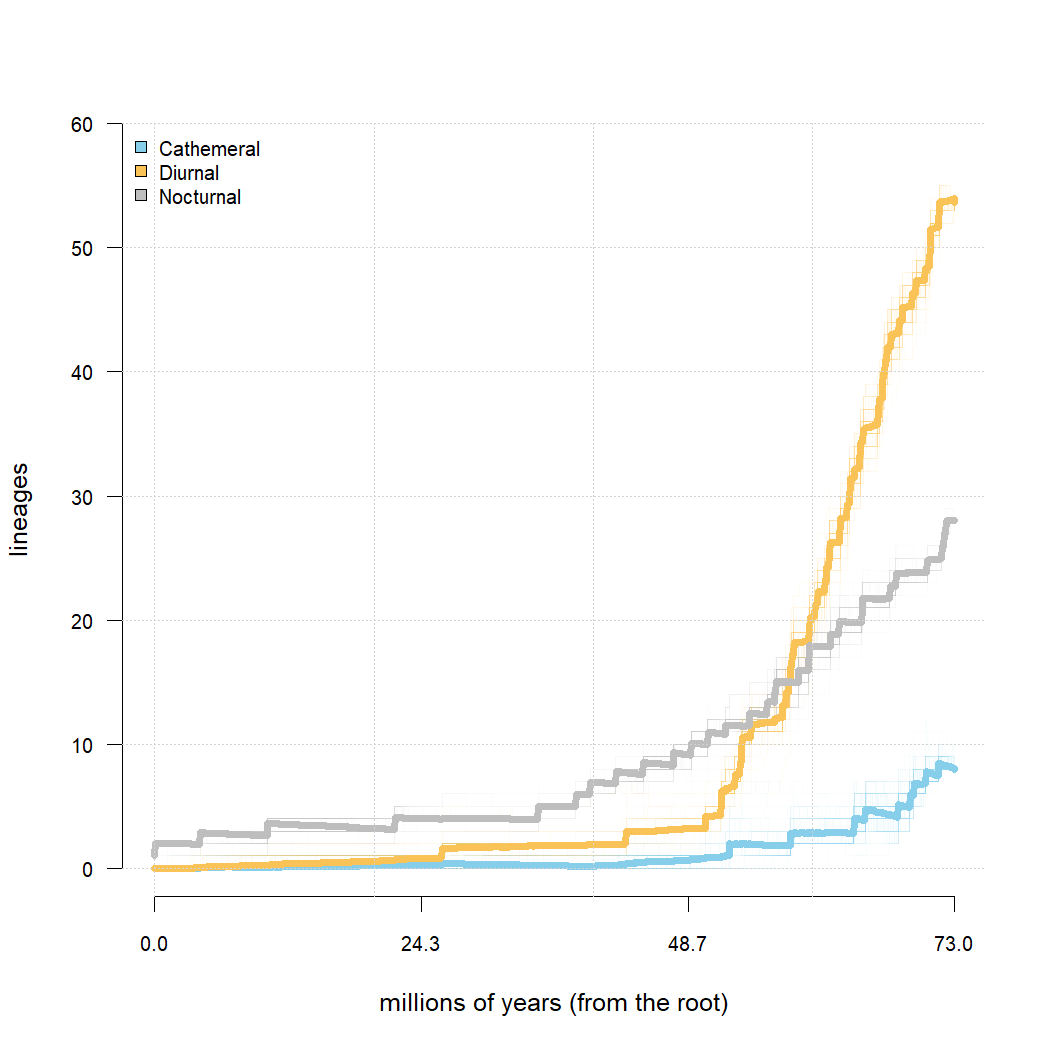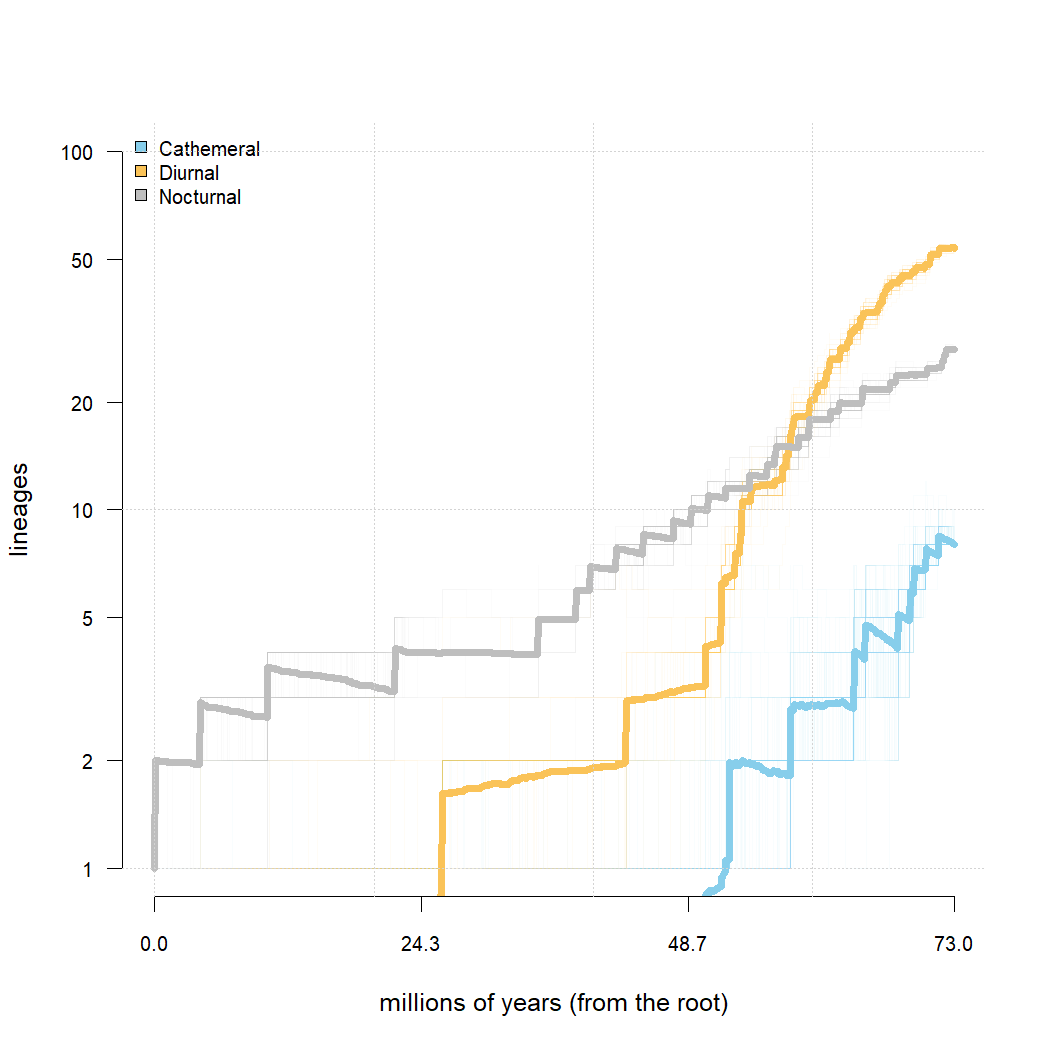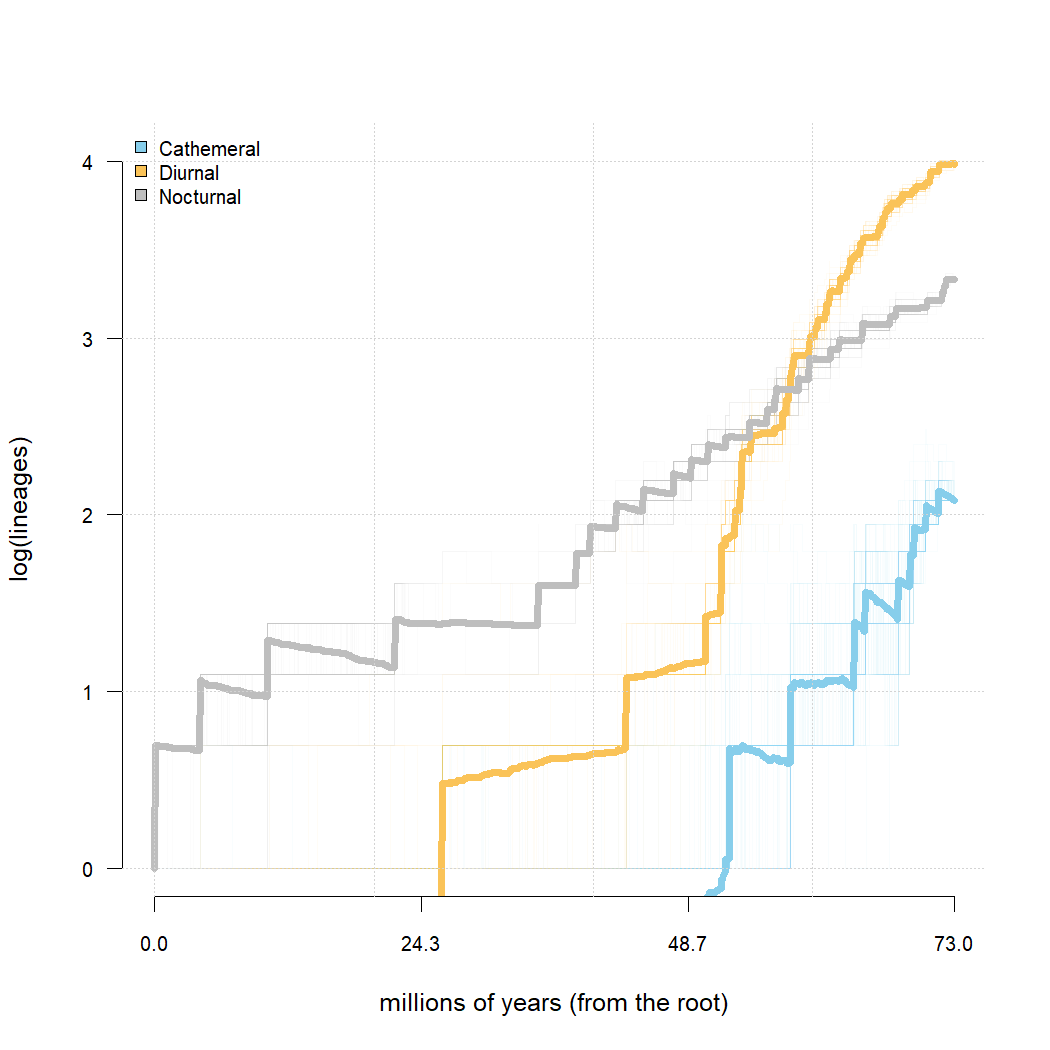## Saturday, December 3, 2022

### LTT for a `"multiSimmap"` object on multiple scales

Today I received the following pleasant email from a phytools user:

“I’m sorry to disturb you, but I had some problems when using the phytools package. I had the honor to read your blog on Monday, August 1, 2022, titled ‘LTT plots for a “multiSimmap” object’. It’s useful for me because it can show lineage accumulation of species with different traits. What I want to know is that how to transfer the values of y axis to the logarithm form? Maybe it could show better variation of accumulation rate? Wish you a happy life! Looking forward to your reply.”

This is pretty easy, but since the question could be of general interest, I thought I’d re-iterate it here, instead of via email.

To do so, I’ll just repeat the same analysis of my previous post.

``````## load packages
library(phytools)
row.names=1,stringsAsFactors=TRUE)
## extract character
Activity_pattern<-setNames(primate.data\$Activity_pattern,
rownames(primate.data))
## run stochastic mapping
primate.maps<-make.simmap(primate.tree,Activity_pattern,model="ARD",
pi="fitzjohn",nsim=100)
``````
``````## make.simmap is sampling character histories conditioned on
## the transition matrix
##
## Q =
##              Cathemeral      Diurnal    Nocturnal
## Cathemeral -0.050529521  0.050529521  0.000000000
## Diurnal     0.002306398 -0.004367451  0.002061052
## Nocturnal   0.002586387  0.003565036 -0.006151422
## (estimated using likelihood);
## and (mean) root node prior probabilities
## pi =
##  Cathemeral     Diurnal   Nocturnal
## 0.003675779 0.021703763 0.974620458
``````
``````## Done.
``````
``````## compute lineage through time plots
primate.ltts<-ltt(primate.maps)
primate.ltts
``````
``````## 100 objects of class "ltt.simmap" in a list
``````

Now we’re ready to proceed with our visualization.

To start, I’ll just repeat the same (non log-scaled) graph that I created in my previous post.

``````## set colors
cols<-setNames(c("#87CEEB","#FAC358","gray"),
levels(Activity_pattern))
## create graph
plot(primate.ltts,colors=cols,show.total=FALSE,bty="n",cex.axis=0.8,
las=1,xlab="millions of years (from the root)",axes=FALSE)
axis(1,at=round(seq(0,max(nodeHeights(primate.tree)),length.out=4),1),
cex.axis=0.8)
axis(2,las=1,cex.axis=0.8)
grid()
``````Next, let’s do this again – but this time I will adjust the generic plotting argument `log="y"`.

This keeps our data on the original scale, but transforms the axis of our plot. Personally, I think this is the best way to visualize data on a logarithmic scale.

``````plot(primate.ltts,colors=cols,show.total=FALSE,
xlim=c(0,max(nodeHeights(primate.tree))),
ylim=c(1,100),bty="n",cex.axis=0.8,
las=1,xlab="millions of years (from the root)",
ylab="lineages",
axes=FALSE,log="y")
axis(1,at=round(seq(0,max(nodeHeights(primate.tree)),
length.out=4),1),cex.axis=0.8)
axis(2,las=1,cex.axis=0.8)
grid()
``````Finally, we can show our number of lineages on a log scale using the LTT specific argument, `log.lineages=TRUE`. Obviously we should not combine `log.lineages=TRUE` with `log="y"`!

``````plot(primate.ltts,colors=cols,show.total=FALSE,
xlim=c(0,max(nodeHeights(primate.tree))),bty="n",
cex.axis=0.8,las=1,xlab="millions of years (from the root)",
axes=FALSE,log.lineages=TRUE)
axis(1,at=round(seq(0,max(nodeHeights(primate.tree)),
length.out=4),1),cex.axis=0.8)
axis(2,las=1,cex.axis=0.8)
grid()
``````Hopefully this addresses the user question!

Note that unlike a standard LTT plot, for this kind of LTT plot the average number of lineages in any given state can drop below one (i.e., have a log value below zero). This means that we need to decide the range of our vertical axis – here I have chosen 0 up to the number of tips in the tree, but this is arbitrary.

That’s it.

#### 1 comment:

1.Thank you for this, it's extremely useful! However, it seems not to work on simmaps made using ambiguous characters. Am I missing something obvious?

Note: due to the very large amount of spam, all comments are now automatically submitted for moderation.